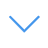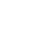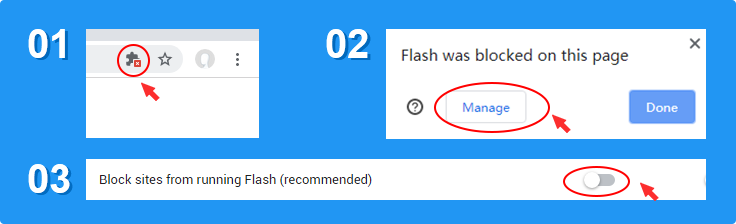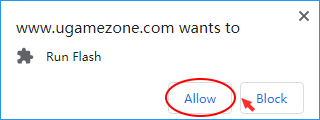# Solve Math

Solve Math is one of the Math Games that you can play on UGameZone.com for free. Are you sensitive to mathematical calculations? This game challenges the player's responsiveness and mathematical computing skills. You have to solve the equals answer by given random numbers and mathematical symbols.

Read MorePress 【Esc】 to Exit Fullscreen

# Solve Math

Solve Math is one of the Math Games that you can play on UGameZone.com for free. Are you sensitive to mathematical calculations? This game challenges the player's responsiveness and mathematical computing skills. You have to solve the equals answer by given random numbers and mathematical symbols.To play the game, you need to allow Adobe Flash Player to run in your browser.

You can use UGameZone Flash Enabler plugin to enable Flash automatically. Or you can follow our instructions to enable Flash manually.

Play Automatically

## Game Ratings

75%

Plays 500

Rating 75%

700
0
Category
Math Games
Tags
Description

Solve Math is one of the Math Games that you can play on UGameZone.com for free. Are you sensitive to mathematical calculations? This game challenges the player's responsiveness and mathematical computing skills. You have to solve the equals answer by given random numbers and mathematical symbols.

Step 1
Step 2